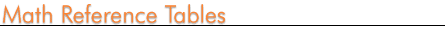Home    |    Teacher    |    Parents    |    Glossary    |    About UsEmail this page to a friendResources· Cool Tools · Formulas & Tables · References · Test Preparation · Study Tips · Wonders of MathSearch( English | Español )---- select language
 General Math Number Notation Addition Table Multiplication Table Interest Constants Fraction-Decimal Conversion Units & Measurement Conversion Algebra Basic Identities Conic Sections Polynomials Exponents Algebra Graphs Functions Geometry Polygon Properties NEW! Area Formulas Volume Formulas Surface Area Formulas Circles - Definitions & Formulas Perimeter Formulas Miscellaneous Vectors Vector Definitions Functions Complexity Graphs Trigonometry Identities Tables Hyperbolas Graphs Functions Calculus Integrals Derivatives Series Expansions English-Spanish Math Dictionary Statistics Distribution Advanced Topics Fourier Series Transforms Recursive Formulas Used with permission from Dave's Math Tables Formula Derivations - (High School +) Derivations of area, perimeter, volume and more for 2 and 3 dimensional figures. (Math Forum)